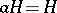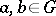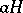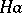# Coset in a group

(diff) ← Older revision | Latest revision (diff) | Newer revision → (diff)by a subgroup(from the left)

A set of elements ofof the formwhereis some fixed element of. This coset is also called the left coset byindefined by. Every left coset is determined by any of its elements.if and only if. For allthe cosetsandare either equal or disjoint. Thus,decomposes into pairwise disjoint left cosets by; this decomposition is called the left decomposition ofwith respect to. Similarly one defines right cosets (as sets,) and also the right decomposition ofwith respect to. These decompositions consist of the same number of cosets (in the infinite case, their cardinalities are equal). This number (cardinality) is called the index of the subgroupin. For normal subgroups, the left and right decompositions coincide, and in this case one simply speaks of the decomposition of a group with respect to a normal subgroup.